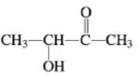# Biacetyl and acetoin are added to margarine to make it taste more like butter. Biacetyl Acetion Complete the Lewis strucrures, predict vnlues for nil C—C—O bond angles, and give the hybridization of the carbon atoms in these two compounds. Must the four carbon atoms and two oxygen atoms in biacetyl lie in the same plane? How many σ bonds and how many π bonds are there in biacetyl and acetoin?### Chemistry: An Atoms First Approach

2nd Edition
Steven S. Zumdahl + 1 other
Publisher: Cengage Learning
ISBN: 9781305079243

#### Solutions

Chapter
Section### Chemistry: An Atoms First Approach

2nd Edition
Steven S. Zumdahl + 1 other
Publisher: Cengage Learning
ISBN: 9781305079243
Chapter 4, Problem 55E
Textbook Problem
3 views

## Biacetyl and acetoin are added to margarine to make it taste more like butter.BiacetylAcetionComplete the Lewis strucrures, predict vnlues for nil C—C—O bond angles, and give the hybridization of the carbon atoms in these two compounds. Must the four carbon atoms and two oxygen atoms in biacetyl lie in the same plane? How many σ bonds and how many π bonds are there in biacetyl and acetoin?

Interpretation Introduction

Interpretation: The structures of two compounds, Biacetyl and Acetoin, have been given. The Lewis structures for these compounds, the values for all CCO bond angles and the hybridization of the carbon atoms present in these compounds is to be predicted. The planar nature of the atoms present in biacetyl and the number of sigma and pi bonds present in both the given compounds is to be determined.

Concept introduction: The hybridization of an atom can be obtained by finding its steric number. The sum of the numbers of atoms bonded to the required atom and the number of lone pairs the atom has is known as the steric number.

If the steric number is 4 , the atom is sp3 hybridized.

If the steric number is 3 , the atom is sp2 hybridized.

If the steric number is 2 , the atom is sp hybridized.

Sigma bonds are the single bonds present between atoms in a compound. The second bonding that occurs between the atoms is known as the pi bonding.

To determine: The Lewis structure, the CCO bond angle value, hybridization of the carbon atoms and the number of sigma and pi bonds present in both the given compounds.

### Explanation of Solution

The electronic configuration of the elements present in the structure of acetoin,

C=1s22s22p2O=1s22s22p4H=1s1

Hydrogen has only one electron. The number of valence electrons in carbon is four and in oxygen is six.

The total numbers of valence electrons present in acetoin are,

4C+8H+2O=((4×4)+(8×1)+(2×6))e=36e

The Lewis structure for acetoin is,

### Still sussing out bartleby?

Check out a sample textbook solution.

See a sample solution

#### The Solution to Your Study Problems

Bartleby provides explanations to thousands of textbook problems written by our experts, many with advanced degrees!

Get Started

Find more solutions based on key concepts
Vitamin D functions as a hormone to help maintain bone integrity. T F

Nutrition: Concepts and Controversies - Standalone book (MindTap Course List)

8-13 Define (a) an Arrhenius acid and (b) an Arrhenius base.

Introduction to General, Organic and Biochemistry

What structural features characterize the prostaglandins?

Chemistry for Today: General, Organic, and Biochemistry

What is hereditarianism, and what is the invalid assumption it makes?

Human Heredity: Principles and Issues (MindTap Course List)

A thin rod of length h and mass M is held vertically with its lower end resting on a frictionless, horizontal s...

Physics for Scientists and Engineers, Technology Update (No access codes included)